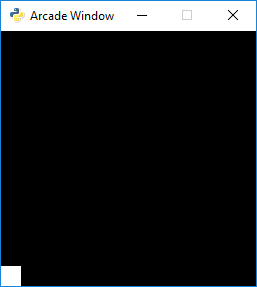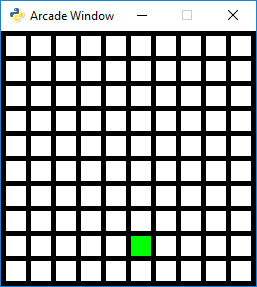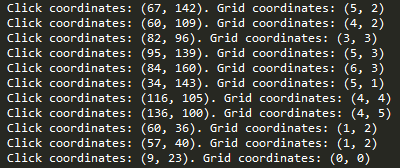# 25. Array-Backed Grids¶

## 25.1. Introduction¶

Games like minesweeper, tic-tac-toe, and many types of adventure games keep data for the game in a grid of numbers. For example, a tic-tac-toe board:

 O O X X

… can use a grid of numbers to represent the empty spots, the O’s and the X’s like this:

 0 2 2 0 1 0 1 0 0

This grid of numbers can also be called a two-dimensional array or a matrix. (Finally, we get to learn about The Matrix.) The values of the numbers in the grid represent what should be displayed at each board location. In the prior example, 0 represents a spot where no one has played, a 1 represents an X, and a 2 represents an O.Figure 16.1: Minesweeper game, showing the backing grid of numbers

The figure above is an example from the classic minesweeper game. This example has been modified to show both the classic display on the left, and the grid of numbers used to display the board on the right.

The number 10 represents a mine, the number 0 represents a space that has not been clicked, and the number 9 represents a cleared space. The numbers 1 to 8 represent how many mines are within the surrounding eight squares, and is only filled in when the user clicks on the square.

Minesweeper can actually have two grids. One for the regular display, and a completely separate grid of numbers that will track if the user has placed “flags” on the board marking where she thinks the mines are.

Classic adventure game maps are created using a tiled map editor. These are huge grids where each location is simply a number representing the type of terrain that goes there. The terrain could be things like dirt, a road, a path, green grass, brown grass, and so forth. Programs like Tiled shown in the figure below allow a developer to easily make these maps and write the grid to disk.Figure 16.2: Using Tiled Map Editor to create an adventure map

Adventure games also use multiple grids of numbers, just like minesweeper has a grid for the mines, and a separate grid for the flags. One grid, or “layer,” in the adventure game represents terrain you can walk on; another for things you can’t walk on like walls and trees; a layer for things that can instantly kill you, like lava or bottomless pits; one for objects that can be picked up and moved around; and yet another layer for initial placement of monsters.

Maps like these can be loaded in a Python program, which is shown in more detail with the Python Arcade Library documentation.

## 25.2. Application¶

Enough talk, let’s write some code. This example will create a grid that will trigger if we display a white or green block. We can change the grid value and make it green by clicking on it. This is a first step to a grid-based game like minesweeper, battleship, connect four, etc. (One year I had a student call me over and she had modified a program like this to show my name in flashing lights. That was … disturbing. So please use this knowledge only for good!)

array_backed_grid.py
  1 2 3 4 5 6 7 8 9 10 11 12 13 14 15 16 17 18 19 20 21 22 23 24 25 26 27 28 29 30 31 32 33 34 35 36 37 38 import arcade SCREEN_WIDTH = 500 SCREEN_HEIGHT = 600 class MyGame(arcade.Window): """ Main application class. """ def __init__(self, width, height): super().__init__(width, height) arcade.set_background_color(arcade.color.WHITE) def on_draw(self): """ Render the screen. """ arcade.start_render() def on_mouse_press(self, x, y, button, key_modifiers): """ Called when the user presses a mouse button. """ pass def main(): window = MyGame(SCREEN_WIDTH, SCREEN_HEIGHT) arcade.run() if __name__ == "__main__": main() 

Starting with the file above, attempt to recreate this program following the instructions here. The final program is at the end of this chapter but don’t skip ahead and copy it! If you do that you’ll have learned nothing. Anyone can copy and paste the code, but if you can recreate this program you have skills people are willing to pay for. If you can only copy and paste, you’ve wasted your time here.

### 25.2.1. Drawing the Grid¶

1. Create variables named WIDTH, HEIGHT, and MARGIN. Set the width and height to 20. This will represent how large each grid location is. Set the margin to 5. This represents the margin between each grid location and the edges of the screen. Create these variables at the top of the program, after the import statements. Also create variables ROW_COUNT and COLUMN_COUNT. Set them to 10. This will control how many rows and columns we will have.
2. Calculate SCREEN_WIDTH and SCREEN_HEIGHT based on the variables we created above. If we have 10 rows, and each row is 20 high, that’s 200 pixels. If we have 10 rows, that’s also 11 margins. (Nine between the cells and two on each edge.) That is 55 more pixels for a total of 255. Write the equation so it works with whatever we select in the constants created by step 1.
3. Change the background to black. Draw a white box in the lower-left corner. Draw the box drawn using the height and width variables created earlier. (Feel free to adjust the colors.) Use the draw_rectangle_filled function. You will need to center the rectangle not at (0, 0) but at a coordinate that takes into account the height and width of the rectangle, such as $$\frac{width}{2}$$. When you get done your program’s window should look like:Figure 16.3: Step 3

1. Use a for loop to draw COLUMN_COUNT boxes in a row. Use column for the variable name in the for loop. The output will look like one long box until we add in the margin between boxes. See Figure 16.4.Figure 16.4: Step 4

1. Adjust the drawing of the rectangle to add in the MARGIN variable. Now there should be gaps between the rectangles. See Figure 16.5.Figure 16.5: Step 5

1. Add the margin before drawing the rectangles, in addition to between each rectangle. This should keep the box from appearing right next to the window edge. See Figure 16.6. You’ll end up with an equation like: $$(margin+width)\cdot column+margin+\frac{width}{2}$$Figure 16.6: Step 6

1. Add another for loop that also will loop for each row. Call the variable in this for loop row. Now we should have a full grid of boxes. See Figure 16.7.Figure 16.7: Step 7

### 25.2.2. Populating the Grid¶

1. Now we need to create a two-dimensional array. We need to create this once, at program start-up. So this will go in the program’s __init__ method. Creating a two-dimensional array in Python is, unfortunately, not as easy as it is in some other computer languages. There are some libraries that can be downloaded for Python that make it easy (like numpy), but for this example they will not be used. To create a two-dimensional array and set an example, use the code below:
Create a 10x10 array of numbers
ROW_COUNT = 10
COLUMN_COUNT = 10

# --- Create grid of numbers
# Create an empty list
self.grid = []
# Loop for each row
for row in range(ROW_COUNT):
# For each row, create a list that will
# represent an entire row
self.grid.append([])
# Loop for each column
for column in range(COLUMN_COUNT):
# Add a the number zero to the current row
self.grid[row].append(0)


A much shorter example is below, but this example uses some odd parts of Python that I don’t bother to explain in this book:

Create a 10x10 array of numbers
self.grid = [[0 for x in range(10)] for y in range(10)]


Use one of these two examples and place the code to create our array ahead of your main program loop.

1. Set an example location in the array to 1.

Two dimensional arrays are usually represented addressed by first their row, and then the column. This is called a row-major storage. Most languages use row-major storage, with the exception of Fortran and MATLAB. Fortran and MATLAB use column-major storage.

# Set row 1, column 5 to one
self.grid = 1


1. Select the color of the rectangle based on the value of a variable named color. Do this by first finding the line of code where the rectangle is drawn. Ahead of it, create a variable named color and set it equal to white. Then replace the white color in the rectangle declaration with the color variable.
2. Select the color based on the value in the grid. After setting color to white, place an if statement that looks at the value in grid[row][column] and changes the color to green if the grid value is equal to 1. There should now be one green square. See Figure 16.8.Figure 16.8: Step 11

1. Print “click” to the screen if the user clicks the mouse button. See Mouse Clicks if you’ve forgotten how to do that.
2. Print the mouse coordinates when the user clicks the mouse.
3. Convert the mouse coordinates into grid coordinates. Print those instead. Remember to use the width and height of each grid location combined with the margin. It will be necessary to convert the final value to an integer. This can be done by using int or by using the integer division operator // instead of the normal division operator /. See Figure 16.9.Figure 16.9: Step 14

1. Set the grid location at the row/column clicked to 1. See Figure 16.10.Figure 16.10: Step 15

### 25.2.3. Resulting Program¶

array_backed_grid.py
  1 2 3 4 5 6 7 8 9 10 11 12 13 14 15 16 17 18 19 20 21 22 23 24 25 26 27 28 29 30 31 32 33 34 35 36 37 38 39 40 41 42 43 44 45 46 47 48 49 50 51 52 53 54 55 56 57 58 59 60 61 62 63 64 65 66 67 68 69 70 71 72 73 74 75 76 77 78 79 80 81 82 83 84 85 86 87 88 89 90 91 92 93 94 95 96 97 98 99 100 101 """ Array Backed Grid Show how to use a two-dimensional list/array to back the display of a grid on-screen. """ import arcade # Set how many rows and columns we will have ROW_COUNT = 10 COLUMN_COUNT = 10 # This sets the WIDTH and HEIGHT of each grid location WIDTH = 20 HEIGHT = 20 # This sets the margin between each cell # and on the edges of the screen. MARGIN = 5 # Do the math to figure out our screen dimensions SCREEN_WIDTH = (WIDTH + MARGIN) * COLUMN_COUNT + MARGIN SCREEN_HEIGHT = (HEIGHT + MARGIN) * ROW_COUNT + MARGIN class MyGame(arcade.Window): """ Main application class. """ def __init__(self, width, height): """ Set up the application. """ super().__init__(width, height) # Create a 2 dimensional array. A two dimensional # array is simply a list of lists. self.grid = [] for row in range(ROW_COUNT): # Add an empty array that will hold each cell # in this row self.grid.append([]) for column in range(COLUMN_COUNT): self.grid[row].append(0) # Append a cell arcade.set_background_color(arcade.color.BLACK) def on_draw(self): """ Render the screen. """ # This command has to happen before we start drawing arcade.start_render() # Draw the grid for row in range(ROW_COUNT): for column in range(COLUMN_COUNT): # Figure out what color to draw the box if self.grid[row][column] == 1: color = arcade.color.GREEN else: color = arcade.color.WHITE # Do the math to figure out where the box is x = (MARGIN + WIDTH) * column + MARGIN + WIDTH // 2 y = (MARGIN + HEIGHT) * row + MARGIN + HEIGHT // 2 # Draw the box arcade.draw_rectangle_filled(x, y, WIDTH, HEIGHT, color) def on_mouse_press(self, x, y, button, modifiers): """ Called when the user presses a mouse button. """ # Change the x/y screen coordinates to grid coordinates column = x // (WIDTH + MARGIN) row = y // (HEIGHT + MARGIN) print(f"Click coordinates: ({x}, {y}). Grid coordinates: ({row}, {column})") # Make sure we are on-grid. It is possible to click in the upper right # corner in the margin and go to a grid location that doesn't exist if row < ROW_COUNT and column < COLUMN_COUNT: # Flip the location between 1 and 0. if self.grid[row][column] == 0: self.grid[row][column] = 1 else: self.grid[row][column] = 0 def main(): window = MyGame(SCREEN_WIDTH, SCREEN_HEIGHT) arcade.run() if __name__ == "__main__": main() 

### 25.2.4. Faster Program¶

The program we have above works, but it is slow. If you look at the CPU of your computer, you’ll find the Python program needlessly taking up CPU time when the grid isn’t changing at all.

Normally, our screens update at 60 frames per second, but for many computers they won’t be able to show even that grid at 60 frames per second. Why? Every single frame we are taking our 100 rectangles, loading the four corners of each rectangle, its colors, and telling the graphics card to draw a box. This results in hundreds of calls to the graphics card.

We can off-load the drawing to the graphics card. We can load all the rectangles to the graphics card, tell the graphics card to remember them, and then redraw them with one command.

This makes drawing a two-step process. Load the the graphics card, and then draw. Slightly more complex, but much faster.

If our shapes change, this program will reload all the shapes. There are more efficient ways to do this, but they are also more complex to program, so we’ll skip that for now.

array_backed_grid_2.py
  1 2 3 4 5 6 7 8 9 10 11 12 13 14 15 16 17 18 19 20 21 22 23 24 25 26 27 28 29 30 31 32 33 34 35 36 37 38 39 40 41 42 43 44 45 46 47 48 49 50 51 52 53 54 55 56 57 58 59 60 61 62 63 64 65 66 67 68 69 70 71 72 73 74 75 76 77 78 79 80 81 82 83 84 85 86 87 88 89 90 91 92 93 94 95 96 97 98 99 100 101 102 103 104 105 106 107 108 109 110 111 112 """ Array Backed Grid version 2 Show how to use a two-dimensional list/array to back the display of a grid on-screen. """ import arcade # Set how many rows and columns we will have ROW_COUNT = 10 COLUMN_COUNT = 10 # This sets the WIDTH and HEIGHT of each grid location WIDTH = 20 HEIGHT = 20 # This sets the margin between each cell # and on the edges of the screen. MARGIN = 5 # Do the math to figure out our screen dimensions SCREEN_WIDTH = (WIDTH + MARGIN) * COLUMN_COUNT + MARGIN SCREEN_HEIGHT = (HEIGHT + MARGIN) * ROW_COUNT + MARGIN class MyGame(arcade.Window): """ Main application class. """ def __init__(self, width, height): """ Set up the application. """ super().__init__(width, height) # Create a 2 dimensional array. A two dimensional # array is simply a list of lists. self.grid = [] for row in range(ROW_COUNT): # Add an empty array that will hold each cell # in this row self.grid.append([]) for column in range(COLUMN_COUNT): self.grid[row].append(0) # Append a cell arcade.set_background_color(arcade.color.BLACK) """ Create a list to hold buffered shapes, and load the list """ self.grid_shape_list = None self.create_shapes_from_grid() def create_shapes_from_grid(self): """ This creates a list of buffered shapes, and loads the rectangles into that list for faster display. """ self.grid_shape_list = arcade.ShapeElementList() for row in range(ROW_COUNT): for column in range(COLUMN_COUNT): # Figure out what color to draw the box if self.grid[row][column] == 1: color = arcade.color.GREEN else: color = arcade.color.WHITE # Figure where to put the box x = (MARGIN + WIDTH) * column + MARGIN + WIDTH // 2 y = (MARGIN + HEIGHT) * row + MARGIN + HEIGHT // 2 # Create the box and add to the list rectangle = arcade.create_rectangle_filled(x, y, WIDTH, HEIGHT, color) self.grid_shape_list.append(rectangle) def on_draw(self): """ Render the screen. """ # This command has to happen before we start drawing arcade.start_render() self.grid_shape_list.draw() def on_mouse_press(self, x, y, button, modifiers): """ Called when the user presses a mouse button. """ # Change the x/y screen coordinates to grid coordinates column = x // (WIDTH + MARGIN) row = y // (HEIGHT + MARGIN) print(f"Click coordinates: ({x}, {y}). Grid coordinates: ({row}, {column})") # Make sure we are on-grid. It is possible to click in the upper right # corner in the margin and go to a grid location that doesn't exist if row < ROW_COUNT and column < COLUMN_COUNT: # Flip the location between 1 and 0. if self.grid[row][column] == 0: self.grid[row][column] = 1 else: self.grid[row][column] = 0 self.create_shapes_from_grid() def main(): window = MyGame(SCREEN_WIDTH, SCREEN_HEIGHT) arcade.run() if __name__ == "__main__": main()